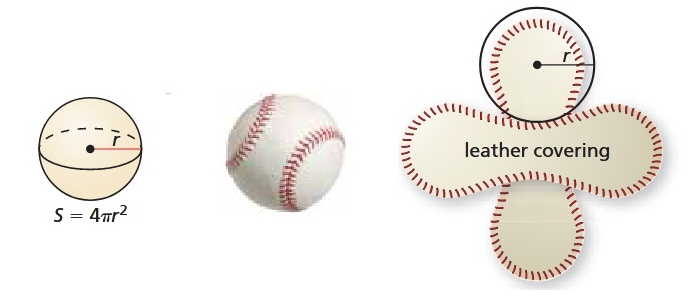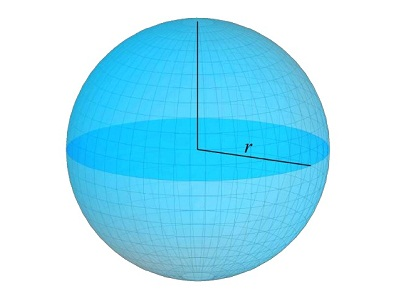# Sphere Surface Area and Volume Calculator

Sphere Calculator is a free online tool used to calculate surface area of sphere and volume of the sphere. Enter the value of radius 'r' of sphere to calculate the area and volume.

## What is a Sphere ?

A sphere is a three-dimensional figure with all points the same distance from a fixed point, the center. A hemisphere is half of a sphere that is created by a plane that intersects a sphere through its center. The edge of a hemisphere is a great circle. The surface area of a sphere is four times the base area, the area of the circle created by the great circle.

A sphere is the set of all points in space equidistant from a given point. This point is called the center of the sphere. A radius of a sphere is a segment from the center to a point on the sphere. A chord of a sphere is a segment whose endpoints are on the sphere. A diameter of a sphere is a chord that contains the center.

### Surface Area of a Sphere Formula

The surface area S of a sphere is:

S = 4πr2

where r is the radius of the sphere.

#### Learn how the Surface Area of Sphere is Calculated

To understand the formula for the surface area of a sphere, think of a baseball. The surface area of a baseball is sewn from two congruent shapes, each of which resembles two joined circles.So, the entire covering of the baseball consists of four circles, each with radius r. The area A of a circle with radius r is A = πr2. So, the area of the covering can be approximated by 4πr2. This is the formula for the surface area of a sphere.

### Volume of the Sphere Formula

The volume V of a sphere is:

V = 4/3 πr3

where r is the radius of the sphere.### Spreading Knowledge Across the World

USA - United States of America  Canada  United Kingdom  Australia  New Zealand  South America  Brazil  Portugal  Netherland  South Africa  Ethiopia  Zambia  Singapore  Malaysia  India  China  UAE - Saudi Arabia  Qatar  Oman  Kuwait  Bahrain  Dubai  Israil  England  Scotland  Norway  Ireland  Denmark  France  Spain  Poland  and  many more....